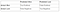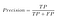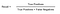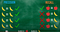# All About Classification Model’s Accuracy — Episode 1Photo by Emily Morter on Unsplash

Hello all, we are going to look at the most confusing concepts related to classification problems in the data science field called the “Confusion Matrix”.

Below is the index of this article.

## Episode 1

1. What is a confusion matrix?

2. What is the accuracy score?

3. Why we need Precession and Recall if we already have Confusion Matrix?

4. What is Precision?

5. What is Recall or Sensitivity or True Positive Rate?

6. What is the F1 Score or Dice similarity coefficient (DSC)?

## Episode 2

7. What is Specificity?

8. What is False Positive Rate?

9. What is False Negative Rate?

10. What is Type — I Error?

11. What is Type — II Error?

11. What is ROC Curve?

12. What is AUC Curve?

# What is Confusion Matrix?

The confusion matrix shows the 4 ways in which our classification model got confused and make predictions.

## Below are four ways.

True Positives (TP): When the classifier correctly predicted that India would win and India did win.

True Negatives (TN): When the classifier correctly predicted that India would not win and India didn’t win.

False Positives (FP): When the classifier incorrectly predicted that India would not win but India ended up winning the match.

False Negatives (FN): When the classifier incorrectly predicted that India would win but India ended up losing the match.CM

# What is Accuracy Score?

It is a number of correct predictions by a total number of predictions.

Below is formula.
accuracy score = (true positives + true negatives) / ( true positives + true negatives + false positives + false negatives )

# Why we need Precession and Recall if we already have a Confusion Matrix?

Sometimes you may prefer a more concise metric.

Below are two short points that will answer this question.

1. One wants to see the true accuracy of the model against the actual positive results.
2. One wants to see the true accuracy of the model against the predicted positive results.

# What is Precision?

Among total positive cases how many of them are positive correct. The higher precision model is good at predicting positive correct in total positive cases.Precision

# What is Recall or Sensitivity or True Positive Rate ?

Among total positive predicted cases how many are positive correct. High recall — model is good at predicting positive correct in total positive predicted cases.Recall

# What is the F1 Score or Dice similarity coefficient (DSC)?

Let’s consider one scenario where the model has high precision in predicting one class and low recall in predicting another class.High Precision and Low Recall

So here we need to balance our accuracy based on this condition so we combine the result of Recall and Precision.

## Two situation

1. F1 Score = 1 indicate perfect precision and the recall.
2. F1 Score = 0 indicate either the precision or the recall is zero.

## Formula

• F1 Score = 2TP / (2TP + FP + FN) Or
• F1 Score = 2 * (precision * recall) / (precision + recall)

## CodeX

Everything connected with Tech & Code

Everything connected with Tech & Code

## More From Medium

Medium is an open platform where 170 million readers come to find insightful and dynamic thinking. Here, expert and undiscovered voices alike dive into the heart of any topic and bring new ideas to the surface. Learn more

Follow the writers, publications, and topics that matter to you, and you’ll see them on your homepage and in your inbox. Explore

If you have a story to tell, knowledge to share, or a perspective to offer — welcome home. It’s easy and free to post your thinking on any topic. Write on Medium